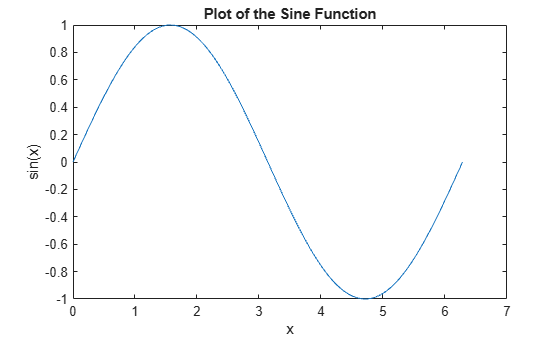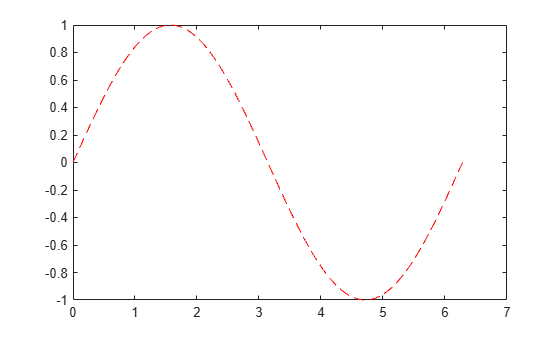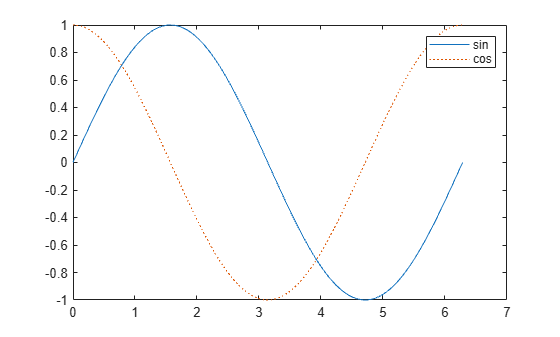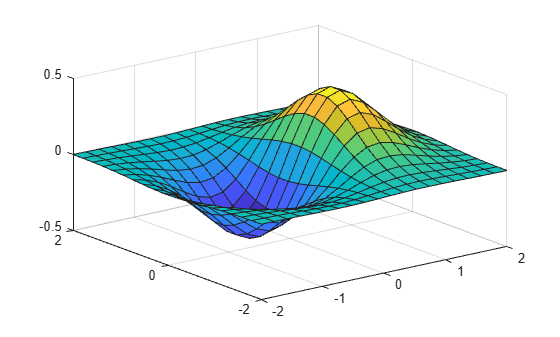# 2-D and 3-D Plots

### Line Plots

To create two-dimensional line plots, use the `plot` function. For example, plot the value of the sine function from 0 to $2\pi$:

```x = 0:pi/100:2*pi; y = sin(x); plot(x,y)```You can label the axes and add a title.

```xlabel('x') ylabel('sin(x)') title('Plot of the Sine Function')```By adding a third input argument to the `plot` function, you can plot the same variables using a red dashed line.

`plot(x,y,'r--')``'r--'` is a line specification. Each specification can include characters for the line color, style, and marker. A marker is a symbol that appears at each plotted data point, such as a `+`, `o`, or `*`. For example, `'g:*'` requests a dotted green line with `*` markers.

Notice that the titles and labels that you defined for the first plot are no longer in the current figure window. By default, MATLAB® clears the figure each time you call a plotting function, resetting the axes and other elements to prepare the new plot.

To add plots to an existing figure, use `hold on`. Until you use `hold off` or close the window, all plots appear in the current figure window.

```x = 0:pi/100:2*pi; y = sin(x); plot(x,y) hold on y2 = cos(x); plot(x,y2,':') legend('sin','cos') hold off```### 3-D Plots

Three-dimensional plots typically display a surface defined by a function in two variables, z = f(x,y) .

To evaluate z, first create a set of (x,y) points over the domain of the function using `meshgrid`.

```[X,Y] = meshgrid(-2:.2:2); Z = X .* exp(-X.^2 - Y.^2);```

Then, create a surface plot.

`surf(X,Y,Z)`Both the `surf` function and its companion `mesh` display surfaces in three dimensions. `surf` displays both the connecting lines and the faces of the surface in color. `mesh` produces wireframe surfaces that color only the lines connecting the defining points.

### Subplots

You can display multiple plots in different subregions of the same window using the `subplot` function.

The first two inputs to `subplot` indicate the number of plots in each row and column. The third input specifies which plot is active. For example, create four plots in a 2-by-2 grid within a figure window.

```t = 0:pi/10:2*pi; [X,Y,Z] = cylinder(4*cos(t)); subplot(2,2,1); mesh(X); title('X'); subplot(2,2,2); mesh(Y); title('Y'); subplot(2,2,3); mesh(Z); title('Z'); subplot(2,2,4); mesh(X,Y,Z); title('X,Y,Z');```